# Units of measurement

(redirected from unit of measurement)
Also found in: Dictionary, Thesaurus, Medical, Financial, Acronyms, Wikipedia.

## Units of measurement

Values, quantities, or magnitudes in terms of which other such are expressed. Units are grouped into systems, suitable for use in the measurement of physical quantities and in the convenient statement of laws relating physical quantities. A quantity is a measurable attribute of phenomena or matter.

A given physical quantity A, such as length, time, or energy, is the product of a numerical value or measure &lcub;A&rcub; and a unit [A]. Thus Eq. (1)

(1)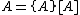holds.

The unit [A] can be chosen arbitrarily, but it is desirable to define units in such a way that they are derived from a few base units by equations without numerical factors other than unity, and that the equations between numerical values of quantities have exactly the same form as the equations between the quantities. For example, the kinetic energy E of a body is given in terms of its mass M and speed V by Eq. (2), (2)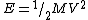where E = [E], M = [M], V = [V], and ½ is called a definitional factor and is dimensionless. If the units of E, M, and V are defined in such a way that Eq. (3) holds, then the equation between the numerical values is Eq. (4).

(3)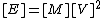(4)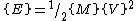A system of units defined in this way is called a coherent system. It is constructed by defining the units of a few base quantities independently; these are called base units. The units of all other quantities are defined by equations similar to Eq. (3) with no numerical factors other than unity, and are called derived units.

In 1960 the General Conference on Weights and Measures (CGPM) gave official status to a single practical system, the International System of Units, abbreviated SI in all languages. The system is a modernized version of the metric system. The SI, as subsequently extended, includes seven base units and twenty-two derived units with special names. These derived units, and others without special names, are derived from the base units in a coherent manner. A set of prefixes is used to form decimal multiples and submultiples of the SI units. Certain units which are not part of the SI but which are widely used or are useful in specialized fields have been accepted for use with the SI or for temporary use in those fields. See Metric system

#### Geometrical units

Units of plane angle and solid angle are purely geometrical. The SI units of plane and solid angle are regarded as dimensionless derived units.

#### Plane angle units

The radian (rad), the SI unit of plane angle, is the plane angle between two radii of a circle which cut off on the circumference an arc equal in length to the radius. Since the circumference of a circle is 2&pgr; times the radius, the complete angle about a point is 2&pgr; rad.

The degree and its decimal submultiples can be used with the SI when the radian is not a convenient unit. By definition, 2&pgr; rad = 360°. The minute [1 = (1/60)°] and the second [1 = (1/60)] can also be used.

#### Steradian

The steradian (sr), the SI unit of solid angle, is the solid angle which, having its vertex at the center of a sphere, cuts off an area on the surface of the sphere equal to that of a square with sides of length equal to the radius of the sphere.

#### Mechanical units

In mechanics, it is convenient to have three base quantities, and two of these are generally chosen to be length and time. Systems of mechanical units may be classified as absolute systems, in which the third base quantity is mass, and gravitational systems, in which the third base quantity is force.

Two absolute systems of metric units are commonly employed, each named for its base units of length, mass, and time: the mks (meter-kilogram-second) absolute system, and the cgs (centimeter-gram-second) absolute system. The mks absolute system is the mechanical portion of the SI. A coherent absolute system of British units is based on the foot, the pound (1 lb ≅ 0.4536 kg), and the second.

Gravitational systems, in which the base quantities are length, force, and time, have been frequently employed by engineers, and are therefore sometimes called technical systems.

#### Length units

The meter (m) is the SI base unit of length. The use of special names for decimal submultiples of the meter should be avoided, and units formed by attaching appropriate SI prefixes to the meter should be used instead.

The angstrom (Å) is equal to 10-10 m. Although it has been accepted for temporary use with the SI, it is preferable to replace this unit with the nanometer, using the relation 1 Å = 0.1 nm.

The nautical mile (nmi), equal to 1852 m, has been accepted for temporary use with the SI in navigation.

The foot (ft) is, as discussed above, the unit of length in the British systems of units, and it is also in customary use in the United States. Since 1959 the foot has been defined as exactly 0.3048 m. The yard (yd) is defined as exactly 3 ft or 0.9144 m. See Length, Measure

Relative measurements of x-ray wavelengths can be made to a higher accuracy than absolute measurements. Before 1965, most x-ray wavelengths were expressed in terms of the X-unit, which is approximately 10-13 m. The X-unit has been superseded by the A* unit, which is based on the tungsten Kα1 line as a standard. The peak of this line is defined as exactly 0.2090100 A*. X-ray wavelength tables have been published in terms of this unit. At the time the A* unit was defined, it was thought to equal 10-10 m (the angstrom unit, Å) to within 5 parts per million, but the A* unit is now believed to be 20 ± 5 parts per million larger than 10-10 m.

Special units whose values are obtained experimentally are used in astronomy. For their definitions.

#### Area units

The square meter (m2), the SI unit of area, is the area of a square with sides of length 1 m. Other area units are defined by forming squares of various length units in the same manner.

Cross sections, which measure the probability of interaction between an atomic nucleus, atom, or molecule and an incident particle, have the dimensions of area, and the appropriate SI unit for expressing them is therefore the square meter. The barn (b), a unit of cross section equal to 10-28 m2, has been accepted for temporary use with the SI.

#### Units of volume

The cubic meter (m3), the SI unit of volume, is the volume of a cube with sides of length 1 m. Other units of volume are defined by forming cubes of various length units in the same manner. The liter (symbol L in the United States) is equal to 1 cubic decimeter (1 dm3), or equivalently to 10-3 m3. It has been accepted for use with the SI for measuring volumes of liquids and gases.

#### Time units

The second (s) is the SI base unit of time. However, other units of time in customary use, such as the minute (1 min = 60 s), hour (1 h = 60 min), and day (1 d = 24 h), are acceptable for use with the SI. See Time

#### Frequency units

The hertz (Hz), the SI unit of frequency, is equal to 1 cycle per second. A periodic oscillation has a frequency of n hertz if it goes through n cycles in 1 s. See Frequency (wave motion)

#### Speed and velocity units

The meter per second (m/s), the SI unit of speed or velocity, is the magnitude of the constant velocity at which a body traverses 1 m in 1 s. Other speed and velocity units are defined by dividing a unit of length by a unit of time in the same manner. See Speed, Velocity

The knot (kn) is equal to 1 nautical mile per hour (1 nmi/h); it has been accepted for temporary use with the SI.

#### Acceleration units

The meter per second squared (m/s2), the SI unit of acceleration, is the acceleration of a body whose velocity changes by 1 m/s in 1 s. Other units of acceleration are defined by dividing a unit of velocity by a unit of time in the same manner. See Acceleration

The gal or galileo (symbol Gal) is equal to 1 cm/s2, or equivalently to 10-2 m/s2.

#### Mass units

The kilogram (kg), the SI base unit of mass, is the only SI unit whose name, for historical reasons, contains a prefix. Names of decimal multiples and submultiples of the kilogram are formed by attaching prefixes to the word gram (g). The metric ton (t), which is equal to 103 kg or 1 megagram (Mg), is permitted in commercial usage of the SI.

The pound (lb), the unit of mass in British absolute system, is also in customary use in the United States. In 1959 the pound was defined to be exactly 0.45359237 kg.

The slug is the unit of mass in the British gravitational system. By definition 1 pound force (lbf) acting on a body of mass 1 slug produces an acceleration of 1 foot per second squared (1 ft/s2). The slug is equal to approximately 32.174 lb or 14.594 kg. See Mass

#### Force units

The newton (N), the SI unit of force, is the force which imparts an acceleration of 1 meter per second squared (1 m/s2) to a body having a mass of 1 kg.

The dyne, the cgs absolute unit of force, is the force which imparts an acceleration of 1 centimeter per second squared (1 cm/s2) to a body having a mass of 1 g.

The unit of force in the British absolute system is the poundal (pdl), the force which imparts an acceleration of 1 foot per second squared (1 ft/s2) when applied to a body of mass 1 lb. One poundal is approximately 0.13825 N.

The units of force in the mks gravitational, cgs gravitational, and British gravitational systems are the forces which impart an acceleration equal to the standard acceleration of gravity, gn = 9.80665 m/s2 ≅ 32.174 ft/s2, when applied to bodies having masses of 1 kg, 1 g, and 1 lb, respectively. These units are named the kilogram force (kgf), gram force (gf), and pound force (lbf), respectively. Unfortunately, these units have also been called simply the kilogram, gram, and pound, giving rise to confusion with the mass units of the same name. See Force

#### Pressure and stress units

The pascal (Pa), the SI unit of pressure and stress, is the pressure or stress of 1 newton per square meter (N/m2). Other units of pressure can also be formed by dividing various units of force by various units of area, such as the pound force per square inch (lbf/in.2, frequently abbreviated psi).

Pressure has been frequently expressed in terms of the bar and its decimal submultiples, where 1 bar = 106 dynes/cm2 = 105 Pa. Pressures are also frequently expressed in terms of the height of a column of either mercury or water which the pressure will support.

Two other units which have been frequently used for measuring pressure are the standard atmosphere and the torr. The standard atmosphere (atm) is exactly 101,325 Pa, which is approximately the average value of atmospheric pressure at sea level. The torr is exactly 1/760 atmosphere, or approximately 133.322 Pa. To within 1 part per million, the torr equal to the pressure of a column of mercury of height 1 millimeter (1 mmHg) at a temperature of 0°C when the acceleration due to gravity has the standard value gn = 9.80665 m/s2. See Pressure, Pressure measurement

#### Energy and work units

The joule (J), the SI unit of energy or work, is the work done by a force of magnitude 1 newton when the point at which the force is applied is displaced 1 m in the direction of the force. Thus, joule is a short name for newton-meter (N-m) of energy or work. See Energy, Work

Units of energy or work in other systems are defined by forming the product of a unit of force and a unit of length in precisely the same manner as in the definition of the joule. Thus, the erg, the cgs absolute unit of energy or work, is the product of 1 dyne and 1 cm.

The foot-poundal (ft-pdl), the British absolute unit of energy or work, is the product of 1 poundal and 1 foot. The foot-pound, or, more properly, the foot-pound force (ft-lbf), the British gravitational unit of energy or work, is the product of 1 lbf and 1 ft.

Sometimes energy is measured in units which are products of a unit of power and a unit of time. Since 1 watt (W) of power equals 1 joule per second (1 J/s), as discussed below, the joule is equivalent to 1 watt-second (1 W · s). In electrical power applications, energy is frequently measured in kilowatthours (kWh), where 1 kWh = (103 W) (3600 s) = 3.6 × 106 J.

The calorie was originally defined as the quantity of heat required to raise the temperature of 1 g of air-free water 1°C under a constant pressure of 1 atm. However, the magnitude of the calorie, so defined, depends on the place on the Celsius temperature scale at which the measurement is made. The International (Steam) Table calorie is defined as exactly 4.1868 J; this is the type of calorie most frequently used in mechanical engineering. The thermochemical calorie, which has been used in thermochemistry in preference to the other types of calorie, is exactly 4.184 J.

The British thermal unit (Btu) was originally defined as the quantity of heat required to raise the temperature of 1 lb of air-free water 1°F under a constant pressure of 1 atm. The International Table Btu is approximately 1055.056 J, and the thermochemical Btu is approximately 1054.350 J.

#### Power units

The watt (W), the SI unit of power, is the power which gives rise to the production of energy at the rate of 1 joule per second (1 J/s). Other units of power can be defined by forming the ratio of a unit of energy to a unit of time in the same manner. See Power

The horsepower (hp) is equal to exactly 550 ft · lbf/s, or approximately 745.700 W.

#### Torque units

The newton-meter (N · m), the SI unit of torque, is the magnitude of the torque produced by a force of 1 newton acting at a perpendicular distance of 1 m from a specified axis of rotation. The joule should never be used as a synonym for this unit.

Units of torque in other systems are defined by forming the product of a unit of force and a unit of length in precisely the same manner as in the definition of the newton-meter.

The foot-poundal (ft · pdl), the British absolute unit of torque, is the product of 1 poundal and 1 foot. The foot-pound (ft · lbf), the British gravitational unit of torque, is the product of 1 lbf and 1 ft. These units are sometimes called the poundal-foot (pdl · ft) and pound-foot (lbf · ft) to distinguish them from the units of energy or work. See Torque

#### Electrical units

For a general discussion of electrical units, including the SI or mks system, three cgs systems [electrostatic system of units (esu), electromagnetic system of units (emu), and gaussian system], and definitions of the SI units ampere (A), volt (V), ohm (&OHgr;), coulomb (C), farad (F), henry (H), weber (Wb), and tesla (T) See Electrical units and standards.

This section discusses some additional SI units and some units in the cgs electromagnetic system which are frequently encountered in scientific literature in spite of the fact that their use has been discouraged.

#### Siemens

The siemens (S), the SI unit of electrical conductance, is the electrical conductance of a conductor in which a current of 1 ampere is produced by an electric potential difference of 1 volt. See Electrical resistance

The siemens was formerly called the mho (&Ogr;) to illustrate the fact the unit is the reciprocal of the ohm.

#### Abampere

The abampere (abA), the cgs electromagnetic unit of current, is that current which, if maintained in two straight, parallel conductors of infinite length, of negligible circular cross section, and placed 1 cm apart in vacuum, would produce between these conductors a force equal to 2 dynes per centimeter of length. The abampere is equal to exactly 10 A.

#### Abvolt

The abvolt (abV), the cgs electromagnetic unit of electrical potential difference and electromotive force, is the difference of electrical potential between two points of a conductor carrying a constant current of 1 abA, when the power dissipated between these points is equal to 1 erg per second. Then 1 abV = 10-8 V.

#### Maxwell

The maxwell (Mx), the cgs electromagnetic unit of magnetic flux, is the magnetic flux which, linking a circuit of one turn, produces in it an electromotive force of 1 abV as it is reduced to zero in 1 s. Then 1 maxwell = 10-8 weber.

#### Units of magnetic flux density

The gauss (Gs), the cgs electromagnetic unit of magnetic flux density (also called magnetic induction), is a magnetic flux density of 1 maxwell per square centimeter (1 Mx/cm2). Then 1 gauss = 10-4 tesla.

#### Units of magnetic field strength

The SI unit of magnetic field strength is 1 ampere per meter (1 A/m), which is the magnetic field strength at a distance of 1 m from a straight conductor of infinite length and negligible circular cross section which carries a current of 2&pgr; A. This definition is based on the definition, in the SI, of the magnetic field strength HSI. At a distance r from a long straight conductor carrying I, HSI is given by Eq. (5).

(5)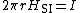The left-hand side of this equation is the line integral of HSI around a circular path, all of whose points are at distance r from the conductor. Substituting r = 1 m, and I = 2&pgr; A in this equation gives HSI = 1 A/m.

The oersted (Oe), the cgs electromagnetic unit of magnetic field strength, is the magnetic field strength at a distance of 1 cm from a straight conductor of infinite length and negligible circular cross section which carries a current of 0.5 abA.

#### Units of magnetic potential and mmf

The ampere serves as the SI unit of magnetic potential difference and magnetomotive force (mmf), as well as the unit of current. In the SI, the magnetomotive force around a closed path equals the current passing through a surface enclosed by the path. Thus, 1 A is the magnetomotive force around a closed path when a current of 1 A passes through an enclosed surface.

The gilbert (Gb), the cgs electromagnetic unit of magnetic potential difference and magnetomotive force, is the magnetomotive force around a closed path enclosing a surface through which flows a current of (1/4&pgr;) abA.

#### Photometric units

Photometric units involve a new base quantity, luminous intensity. For the definition of the candela (cd), the SI unit of luminous intensity, See Photometry, Physical measurement

#### Lumen

The lumen (lm), the SI unit of luminous flux, is the luminous flux emitted within a unit solid angle (1 steradian) by a point source having a uniform intensity of 1 candela. See Luminous flux

#### Luminous energy units

The lumen-second (lm·s), the SI unit of luminous energy (also called quantity of light), is the luminous energy radiated or received over a period of 1 s by a luminous flux of 1 lumen. This unit is also called the talbot. See Luminous energy

#### Radiation units

Certain quantities and units are used particularly in the area of ionizing radiation. The special units curie, roentgen, rad, and rem, which were previously adopted for use in this area, are not coherent with the SI, but their temporary use with the SI has been approved while the transition to SI units takes place.

#### Activity units

The becquerel (Bq), the SI unit of activity (radioactive disintegration rate), is the activity of a radionuclide decaying at the rate of one spontaneous nuclear transition per second. Thus 1 Bq = 1 s-1.

The curie (Ci), the special unit of activity, is equal to 3.7 × 1010 Bq. See Radioactivity

#### Exposure units

The SI unit of exposure to ionizing radiation, 1 coulomb per kilogram (1 C/kg), is the amount of electromagnetic radiation (x-radiation or gamma radiation) which in 1 kg of pure dry air produces ion pairs carrying 1 coulomb of charge of either sign. (The ionization arising from the absorption of bremsstrahlung emitted by electrons is not to be included in measuring the charge.) See Bremsstrahlung

The roentgen (R), the special unit of exposure, is equal to 2.58 × 10-4 C/kg.

#### Absorbed dose units

The gray (Gy), the SI unit of absorbed dose, is the absorbed dose when the energy per unit mass imparted to matter by ionizing radiation is 1 joule per kilogram (1 J/kg).

The rad (rd), the special unit of absorbed dose, is equal to 10-2 Gy.

#### Dose equivalent units

Different types of radiation cause slightly different effects in biological tissue. For this reason, a weighted absorbed dose called the dose equivalent is used in comparing the effects of radiation on living systems. The dose equivalent is the product of the absorbed dose and various dimensionless modifying factors.

The sievert (Sv), the SI unit of dose equivalent, is the dose equivalent when the absorbed dose of ionizing radiation multiplied by the stipulated dimensionless factors is 1 joule per kilogram (1 J/kg).

The rem, the special unit of dose equivalent, is equal to 10-2 Sv.

McGraw-Hill Concise Encyclopedia of Physics. © 2002 by The McGraw-Hill Companies, Inc.
References in periodicals archive ?
To test whether the observed differences are significant we apply a Wilcoxon signed rank test.(7) The effect of changing unit of measurement is analyzed by comparing the weighted patient and the weighted patient day model, while the effect of changing the aggregation criterion is analyzed by comparing the (weighted) patient day model using the surgical/medical distinction and the patient day model using the simple/complex distinction.
When Wales ceases to be the unit of measurement for a disaster, you can be sure that it really is a mega-disaster.
On which part of the human body is the cubit unit of measurement based?
Question: What unit of measurement is used to size up a horse?

Site: Follow: Share:
Open / Close